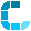# Conversion ... acceleration

Unit of input

Unit of outputSelection input > output units Description In Unit Out kilometers per second squared km/s² meters per second squared m/s² centimeters per second squared cm/s² millimeters per second squared mm/s² standard acceleration unit (gravity) g. mile per sec. squared mi/s² foot per sec. squared ft/s² inch per sec. squared in/s² Gal gal, Gal